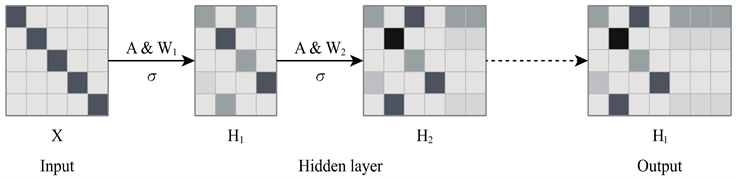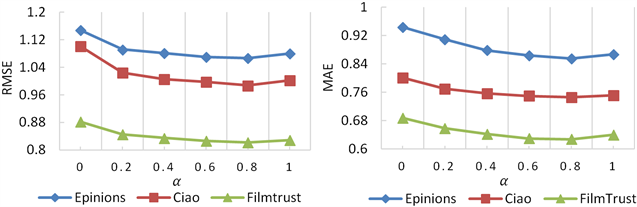# 融合社交网络用户相似度的社会化推荐Social Recommendation with Social Network Users Similarity

DOI: 10.12677/CSA.2021.111003, PDF, HTML, XML, 下载: 109  浏览: 261  科研立项经费支持

Abstract: In view of the low accuracy of traditional social recommendation, a social recommendation algorithm SRSUS integrating social network user similarity was proposed based on the comprehensive consideration of social network user similarity factors, such as subgraph topology, user trust and user rating similarity. The algorithm takes traditional matrix decomposition as the framework. Firstly, the graph convolutional neural network is used to learn the user’s social network to obtain the user’s potential characteristics including the topology structure and connection relations of the social network subgraph. Then social relation is used to calculate user’s social trust and score data is used to calculate the user rating similarity. Finally, user potential characteristics, user trust and user rating similarity are comprehensively used to calculate social network user similarity and then integrate it into user rating matrix decomposition. In this way, the user's rating of the predicted item can be predicted. Experimental results on Epinions, Filmtrust, Ciao and other public data sets show that this algorithm is generally superior to other social recommendation algorithms.

1. 引言

2. 相关工作

3. 社交网络用户相似度计算

3.1. 社交网络用户潜在特征计算Figure 1. Graph convolutional neural network propagation process

${H}^{\left(l+1\right)}=f\left({H}^{\left(l\right)},A\right)=\sigma \left({\stackrel{˜}{D}}^{-\frac{1}{2}}\stackrel{˜}{A}{\stackrel{˜}{D}}^{-\frac{1}{2}}{H}^{\left(l\right)}{W}^{\left(l\right)}\right)$ (1)

$si{m}_{social}\left({U}_{i},{U}_{j}\right)=\frac{\underset{d=1}{\overset{d}{\sum }}{U}_{id}×{U}_{jd}}{\sqrt{\underset{d=1}{\overset{d}{\sum }}{\left({U}_{id}\right)}^{2}}\sqrt{\underset{d=1}{\overset{d}{\sum }}{\left({U}_{jd}\right)}^{2}}}$ (2)

${U}_{id}$${U}_{jd}$ 为用户 ${U}_{i}$${U}_{j}$ 的第d维向量值。

3.2. 社交网络用户信任度计算

$T\left(u,v\right)=\frac{{W}_{u,v}}{f\left({U}_{u},{U}_{v}\right)}$ (3)

$Trust{p}_{i}\left({u}_{i},{v}_{i}\right)=\left(T\left({u}_{i},{a}_{i1}\right)+T\left({a}_{i1},{a}_{i2}\right)+\cdots +T\left({a}_{ij},{v}_{i}\right)\right)×{\text{e}}^{-\left(d-1\right)}$ (4)

$Trust\left(u,v\right)=\frac{{\sum }_{i\in n}Trust{p}_{i}\left({u}_{i},{v}_{i}\right)}{n}$ (5)

3.3. 用户评分相似度计算

${R}_{m×n}=\left(\begin{array}{ccc}{R}_{11}& \dots & {R}_{1n}\\ ⋮& \ddots & ⋮\\ {R}_{m1}& \cdots & {R}_{mn}\end{array}\right)$

$si{m}_{score}\left({U}_{i},{U}_{j}\right)=\frac{\underset{k=1}{\overset{n}{\sum }}{R}_{ik}×{R}_{jk}}{\sqrt{\underset{k=1}{\overset{n}{\sum }}{R}_{ik}^{2}}×\sqrt{\underset{k=1}{\overset{n}{\sum }}{R}_{jk}^{2}}}$ (6)

4. 社会化推荐算法SRSUS

4.1. 算法原理

$L=\frac{1}{2}\underset{i=1}{\overset{m}{\sum }}\underset{j=1}{\overset{n}{\sum }}{I}_{ij}{\left({R}_{ij}-{U}_{i}{V}_{j}^{\text{T}}\right)}^{2}+\frac{{\lambda }_{1}}{2}{‖U‖}_{F}^{2}+\frac{{\lambda }_{2}}{2}{‖V‖}_{F}^{2}\frac{{\lambda }_{3}}{2}\underset{i=1}{\overset{m}{\sum }}\underset{{U}_{j}\in F\left(i\right)}{\sum }sim\left({U}_{i},{U}_{j}\right){‖{U}_{i}-{U}_{j}‖}^{2}$ (7)

$sim\left({U}_{i},{U}_{j}\right)=\alpha si{m}_{social}\left({U}_{i},{U}_{j}\right)+\beta Trust\left({U}_{i},{U}_{j}\right)+\gamma si{m}_{score}\left({U}_{i},{U}_{j}\right)$ (8)

$\frac{\partial L}{\partial {U}_{i}}=\underset{i=1}{\overset{m}{\sum }}{I}_{ij}\left({R}_{ij}-{U}_{i}{V}_{j}^{\text{T}}\right){V}_{j}+{\lambda }_{1}{U}_{i}+{\lambda }_{3}\underset{i=1}{\overset{m}{\sum }}{Z}_{i}×{{Z}^{\prime }}_{i}$ (9)

$\frac{\partial L}{\partial {V}_{j}}=\underset{j=1}{\overset{n}{\sum }}{I}_{ij}\left({R}_{ij}-{U}_{i}{V}_{j}^{\text{T}}\right){U}_{i}+{\lambda }_{2}{V}_{j}$ (10)

4.2. 算法步骤

5. 实验结果与分析

5.1. 数据集Table 1. Dataset information statistics

5.2. 评价指标

$\text{MAE}=\frac{{\sum }_{\left(i,j\right)\in N}|{R}_{ij}-{r}_{ij}|}{|N|}$ (11)

$\text{RMSE}=\sqrt{\frac{{\sum }_{\left(i,j\right)\in N}|{R}_{ij}-{r}_{ij}|}{|N|}}$ (12)

5.3. 实验结果与分析Figure 2. RMSE and MAE values in different parameter $\alpha$Figure 3. RMSE and MAE values in different number of similar usersTable 2. Performance comparison of different algorithms

6. 总结与展望

  Lu, Z.Q., Dou, Z.C., Lian, J.X., et al. (2015) Content-Based Collaborative Filtering for News Topic Recommendation. Proceedings of the 29th Conference on Artificial Intelligence, Austin, 25-30 January 2015, 217-223.  Ji, K., Sun, R., Li, X., et al. (2016) Improving Matrix Approximation for Recommendation via a Clustering-Based Reconstructive Method. Neurocomputing, 173, 912-920. https://doi.org/10.1016/j.neucom.2015.08.046  Pan, J.C., Zhang, X.M. and Wang, X. (2016) Improved Singular Value Decomposition Recommender Algorithm Based on User Reliability. Journal of Chinese Computer System, 37, 2171-2176.  Massa, P. and Bhattacharjee, B. (2004) Using Trust in Recommender Systems: An Experimental Analysis. LNCS 2995: Proceedings of the 2nd International Conference on Trust Management, Oxford, 29 March-1 April 2004, 221-235. https://doi.org/10.1007/978-3-540-24747-0_17  Yang, B., Lei, Y., Liu, D., et al. (2013) Social Collaborative Filtering by Trust. In: Proceedings of the Twenty-Third International Joint Conference on Artificial Intelligence, Morgan Kaufmann, San Francisco, 2747-2753.  Ma, H., Yang, H.X., Lyu, M.R., et al. (2008) SoRec: Social Recommenda-tion Using Probabilistic Matrix Factorization. In: Proceedings of the 17th on Information and Knowledge Management, ACM Press, New York, 978-991. https://doi.org/10.1145/1458082.1458205  Ma, H., King, I. and Lyu, M.R. (2009) Learning Tore Commend with Social Trust Ensemble. Proceedings of the 32nd International ACM SIGIR Conference on Research and Develop-ment in Information Retrieval, Boston, 19-23 July 2009, 203-210. https://doi.org/10.1145/1571941.1571978  Ma, H., Zhou, D., Liu, C., et al. (2011) Recommender Systems with Social Regularization. Forth International Conference on Web Search & Web Data Mining, Hong Kong, 9-12 Feb-ruary 2011, 287-296. https://doi.org/10.1145/1935826.1935877  Zhang, T.W., Li, W.P., Wang, L., et al. (2020) Social Recommen-dation Algorithm Based on Stochastic Gradient Matrix Decomposition in Social Network. Journal of Ambient Intelli-gence and Humanized Computing, 11, 601-608. https://doi.org/10.1007/s12652-018-1167-7  吴清春, 贾彩燕. 一种融合社交关系的矩阵分解推荐模型[J]. 计算机工程, 2020, 46(8): 72-77+84.  Chen, H.X., Yin, H.Z., Chen, T., et al. (2020) Social Boosted Recommenda-tion with Folded Bipartite Network Embedding. IEEE Transactions on Knowledge and Data Engineering. https://doi.org/10.1109/TKDE.2020.2982878  Kipf, T.N. and Welling, M. (2016) Semi-Supervised Classifica-tion with Graph Convolutional Networks. ICLR International Conference on Learning Representations, Toulon, 26-27 April 2017. arXiv:1609.02907.  Berg, R.V.D., Kipf, T.N. and Welling, M. (2017) Graph Convolutional Matrix Completion.  Ying, R., He, R., Chen, K., et al. (2018) Graph Convolutional Neural Networks for Web-Scale Rec-ommender Systems. ACM International Conference on Knowledge Discovery and Data Mining, London, 19-23 August 2018, 974-983. https://doi.org/10.1145/3219819.3219890  He, X.N., Deng, K., Wang, X., et al. (2020) LightGCN: Simplify-ing and Powering Graph Convolution Network for Recommendation. Proceedings of the 43rd International ACM SIGIR Conference on Research and Development in Information, Xi’an, 25-30 July 2020, 639-648. https://doi.org/10.1145/3397271.3401063  赵亮, 陈平华, 廖威平. 融合社交网络用户潜在因子的社会化推荐[J]. 计算机工程与应用, 2020, 56(24): 169-174.  杨鹏, 邵堃, 霍星, 张阳洋, 景永俊. 融合用户隐含偏好的社会化推荐算法[J]. 小型微型计算机系统, 2019, 40(10): 2039-2045.